Time requried to exclude single machine cycle in MC9S12XHZ512

cancel
Showing results for
Did you mean:

Time requried to exclude single machine cycle in MC9S12XHZ512

315 Views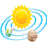Contributor III

Can someone tell me how can I calculate time required by timer to increment by 1, if I am using 16MHz crystal with noprescale in MC9S12XHZ512 .

Labels (1)
• General

7 Replies
116 ViewsNXP TechSupport

Hi KDN,

As for the timer block use the bus clock (according the related registers ),firstly you need calculate the bus clock , then you can get the time of timer increment 1.

Below is the mainly calculate process ( MC9S12XHZ512):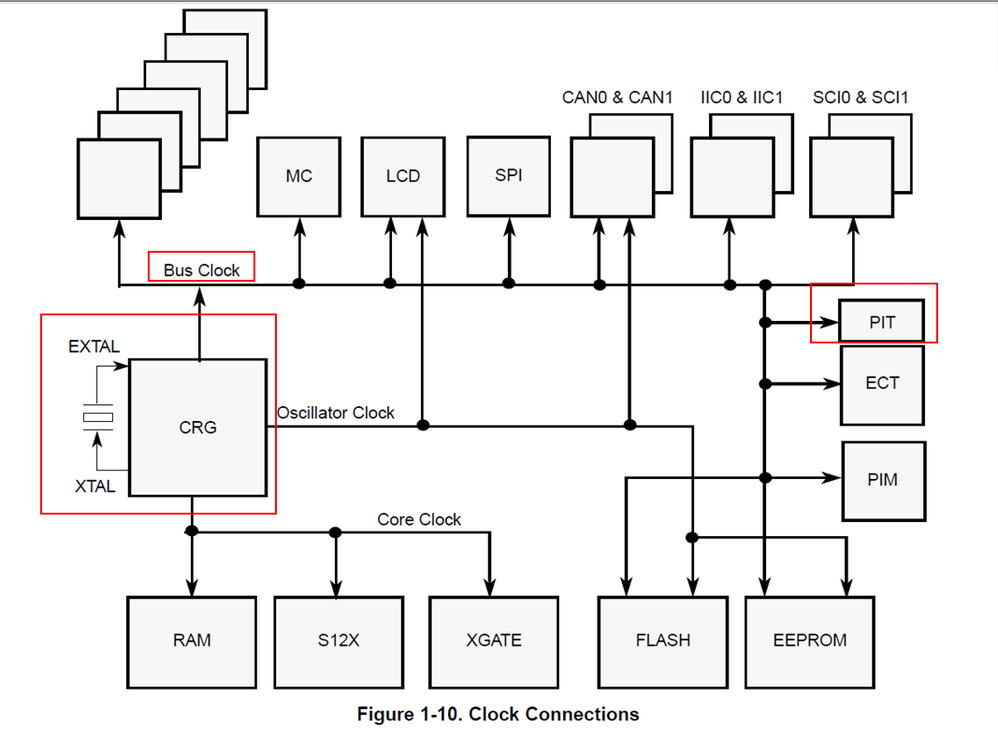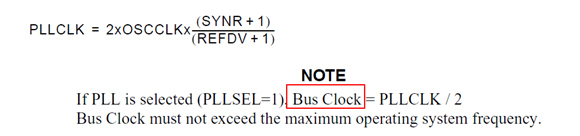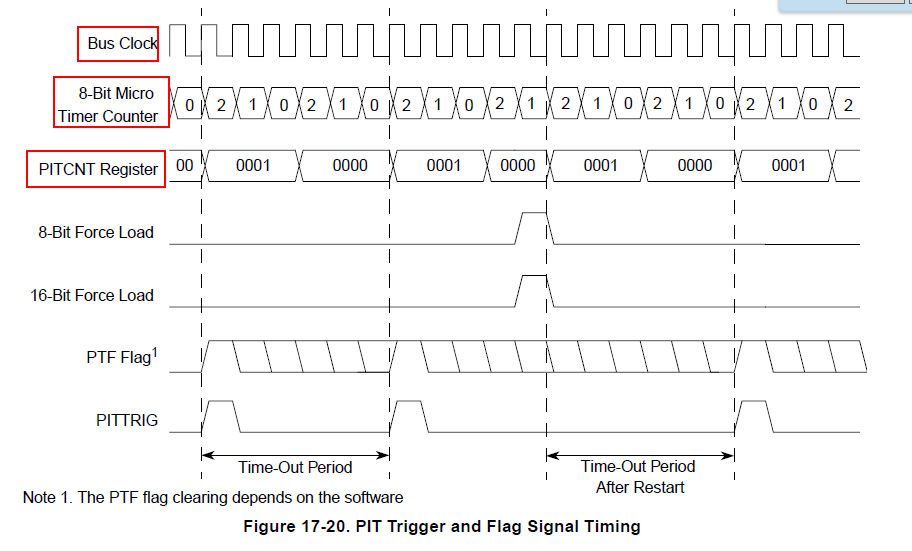You can according your configuration of register and the calculate procedure , get the result.

If you still  have  any question,  welcome to the community discuss.

Alice

116 ViewsContributor III

Thanks for replay , I have not selected PPL.

What actually I want to do , I am using Input capture mode of MC9S12XHZ512 for calculating frequency and duty cycle of square wave from 1KHz to 10KHz , I have attached image of setting i have done in codewarrior.

My problem is that when I try to display frequency on my LCD display I am getting random charter on , I also have problem with interrupt input capture mode I have enable input capture mode in codewarrior , but i'm not able to find it in program , I want to write my display and calculation code in interrupt sub routine of input capture mode. I have posted my code below.

I'm using 16MHz crystal

LCD_Cmd(0x80);

//  TM=65535*(TC0/16000000);

//  TM=1/TM;

t[i]=TC0;

i++;

if(i==2) {

i=0;

time_interval=(t-t);

time_interval=(float)(0.0000000625*time_interval);

frequency= 1/time_interval;

sprintf(buffer,"%d",frequency);

for(j=0;j<5;j++)

{

LCD_Data(buffer[j]);

}

}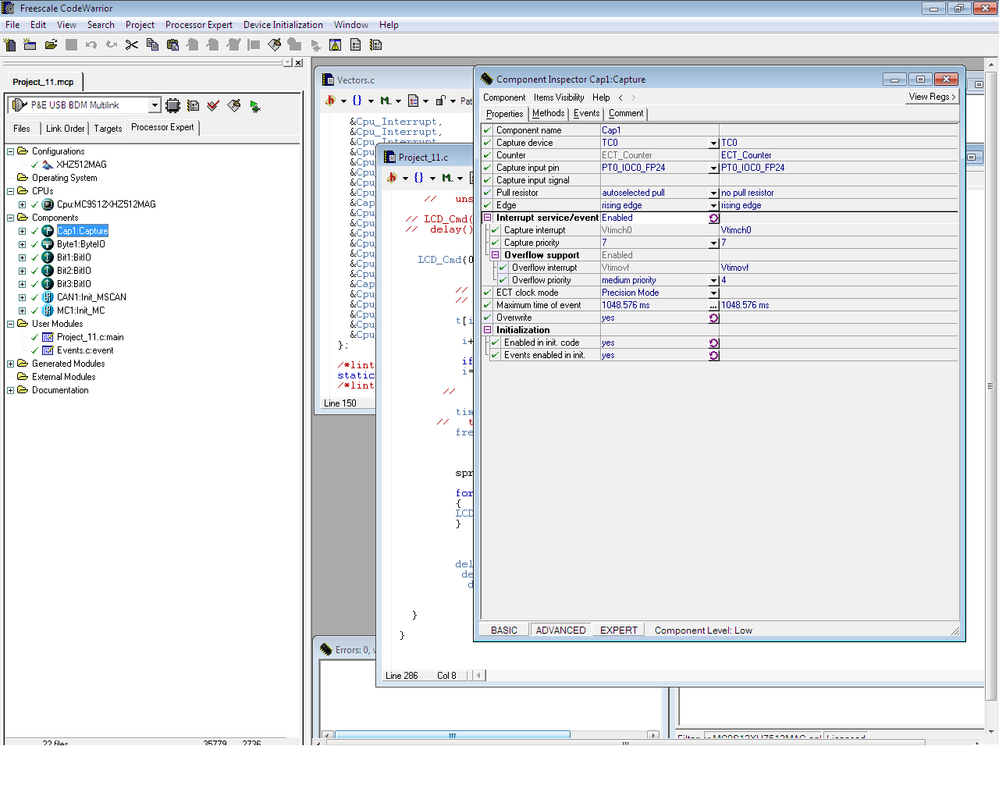116 ViewsNXP TechSupport

Hi KDN,

In your code , you should enable the capture component and interrupt like this :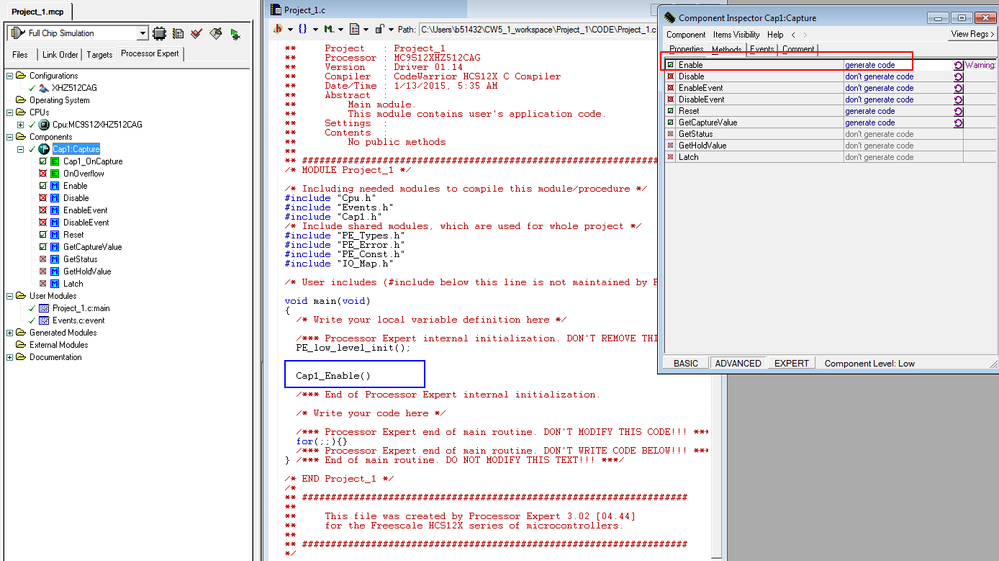Then you test whether it can enter the interrupt .

Alice

116 ViewsContributor III

Thanks for replay , I done that ,

Now my problem is this calculation .

unsigned int time_interval;

unsigned int t,t1,t2;

unsigned int j,k=0;

float  time_period,frequency,value,test;

unsigned char buffer;

t[k]= TCNT;

k++;

if(k==0)

{

time_interval=(t-t);

time_period=(float)(0.000004*time_interval);

frequency= 1/time_period;

sprintf(buffer,"%f",frequency);

for(j=0;j<4;j++)

{

LCD_Data( buffer[j]);

}

}

I am not able to convert from int to float.

116 ViewsNXP TechSupport

Hi KDN,

When you want use float data, when you create project , please choose like this :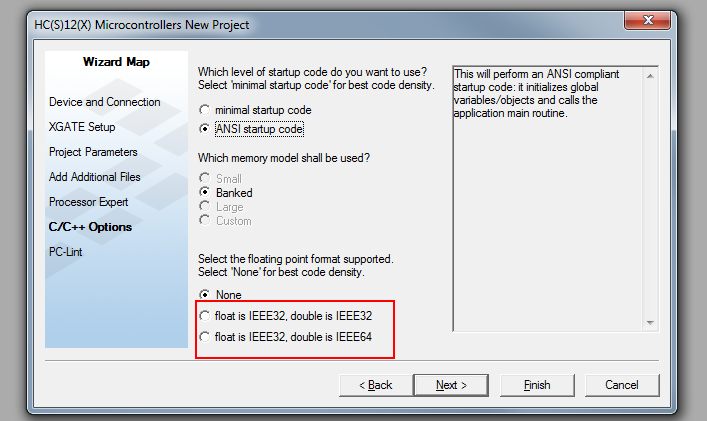Hope it helps

Alice

116 ViewsContributor III

CAN I read 100Khz frequency using MC9S12XHZ512 Micro-controller ?

116 ViewsContributor III

Thanks I Have selected float IEEE32 and double IEEE32

But not working